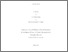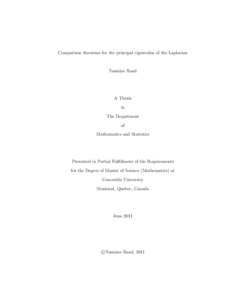Title:

Comparison theorems for the principal eigenvalue of the Laplacian.

Raad, Yasmine (2011) Comparison theorems for the principal eigenvalue of the Laplacian. Masters thesis, Concordia University.Preview
Text (application/pdf)
377kB

Abstract

We study the Faber - Krahn inequality for the Dirichlet eigenvalue problem of the
Laplacian, ﬁrst in \$R^N\$ , then on a compact smooth Riemannian manifold M . For the
latter, we consider two cases. In the ﬁrst case, the compact manifold has a lower bound
on the Ricci curvature, in the second, the integral of the reciprocal of an isoperimetric
estimator function of the Riemannian manifold is convergent. In all cases, we show
that the ﬁrst eigenvalue of a domain in \$R^N\$ , respectively M , is minimal for the ball
of the same volume, respectively, for a geodesic ball of the same relative volume in
an appropriate manifold \$M^∗\$ . While working with the isoperimetric estimator, the
manifold \$M^∗\$ need not have constant sectional curvature. In \$R^N\$ , we also consider the
Neumann eigenvalue problem and present the Szeg¨
o - Weinberger inequality. In this
case, the principal eigenvalue of the ball is maximal among all principal eigenvalues
of domains with same volume.

Divisions: Concordia University > Faculty of Arts and Science > Mathematics and Statistics Thesis (Masters) Yes Raad, Yasmine supervisor, Alina Stancu Concordia University M. Sc. Mathematics September 2011 Stancu, Alina 35889 YASMINE RAAD 21 Nov 2011 20:21 18 Jan 2018 17:35
All items in Spectrum are protected by copyright, with all rights reserved. The use of items is governed by Spectrum's terms of access.

Repository Staff Only: item control page

Back to top